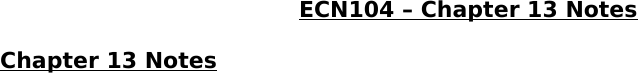Textbook Notes (290,000)
CA (170,000)
Ryerson (10,000)
ECN (800)
ECN 104 (300)
Chapter 13

# ECN 104 Chapter Notes - Chapter 13: Farmer Jack, Average Cost, Marginal Product

Department
Economics
Course Code
ECN 104
Professor
Chapter
13

Page:
of 4ECN104 Chapter 13 Notes
Chapter 13 Notes
Questions
oWhat is a production function? What is marginal product? How are they
related?
oWhat are the various costs, and how are they related to each other and
to output?
oHow are costs different in the short run vs. the long run?
oWhat are the “economics of scale”?
Total Revenue, Total Cost, Profit
oWe assume that the firm’s goal is to maximize profit.
oProfit = Total Revenue (the amount a firm receives from the sale of its
output) – total cost (the market value of the inputs a firm uses in
production)
Costs: Explicit vs. Implicit
oExplicit costs: require an outlay of money
E.g., paying wages to workers
oImplicit costs: do not require a cash outlay
E.g., the opportunity cost of the owner’s time
oRemember one of the Ten Principles:
The cost of something is what you give up to get it
oThis is true whether the costs are implicit or explicit. Both matter for
firms’ decisions
oExample:
Interest rate is 5%
Case 1:
Borrow \$100,000
Explicit cost = \$5000 interest on loan
Case 2:
Use \$40,000 of your savings, borrow the other \$60,000
Explicit cost = \$3000 (5%) interest on the loan
Implicit cost = \$2000 (5%) foregone interest you could
In both cases, total (explicit + implicit) costs equal \$5000
Economic Profit vs. Accounting Profit
oAccounting profit = total revenue – total explicit costs
oEconomic profit = total revenue – total costs (including explicit and
implicit costs)
oAccounting profit ignores implicit costs, so its higher than economic
profit
The Production Function
oShows the relationship between the quantity of inputs used to produce
a good and the quantity of output of that good
oIt can be represented by a table, equation, or graph
oExample:
Farmer Jack grows wheat
He has 5 acres of land
He can hire as many workers as he wants
Marginal Product
oIf Jack hires one more worker, his output rises by the marginal produce
of labour
oThe marginal product of any input is the increase in output arising
from an additional unit of that input, holding all other inputs constant
oMarginal product of labour (MPL) = (Change in output) / (Change in
labour)
Why MPL is Important
oRecall one of the Ten Principles:
Rational people think at the margin.
oWhen Farmer Jack hires an extra worker,
His costs rise by the wage he pays the worker
His output rises by MPL
oComparing them helps Jack decide whether he would benefit from
hiring the worker
Why MPL diminishes
oFarmer Jack’s output rises by a smaller and smaller amount for each
oAs Jack adds workers, the average worker has less land to work with
and will be less productive
oIn general, MPL diminishes as Labour rises whether the fixed input is
land or capital (equipment, machines, etc.)
oDiminishing Marginal Product: the marginal product of an input
declines as the quantity of the input increases (other things equal)
Example 1: Farmer Jack’s Costs
oFarmer Jack must pay \$1000 per month for the land, regardless of how
much wheat he grows
oThe market wage for a farm worker is \$2000 per month
oSo Farmer Jack’s costs are related to how much wheat he produces
Marginal Cost
oIncrease in Total Cost from producing one more unit
oMC = (Change in Total Cost) / (Change in Output)
Why MC is Important
oFarmer Jack is rational and wants to maximize his profit. To increase
profit, should he produce more or less wheat?
oTo find the answer, Farmer Jack needs to “think at the margin.”
oIf the cost of additional wheat (MC) is less than the revenue he would
get from selling it, then Jack’s profits rise if he produces more
Fixed and Variable Costs
oFixed Costs (FC) do not vary with the quantity of output produced
For Farmer Jack, FC = \$1000 for his land
Other examples: cost of equipment, loan payments, rent
oVariable Costs (VC) vary with the quantity produced
For Farmer Jack, VC = wages he pays workers
Other example: cost of materials
oTotal Cost (TC) = FC + VC
oAverage Total Cost (ATC) equals total cost divided by the quantity
of output
ATC = TC/Q
ATC = Average FC + Average VC
oEfficient Scale: the quantity that minimizes ATC
oExample:
Suppose PPG (points per game) of Kobe Bryant is 30.0
If he scores more than 30 in tonight’s game, his PPG will
increase
If he scores less than 30 in tonight’s game, his PPG will decrease
His PPG corresponds to ATC, tonight’s score corresponds to MC
Costs in the Short Run & Long Run
oShort run:
Some inputs are fixed (e.g., factories, land)
The costs of these inputs are FC
oLong run:
All inputs are variable
E.g., firms can build more factories or sell existing ones
oIn the long run, ATC at any Output is cost per unit using the most
efficient mix of inputs for that Output (e.g., the factory size with the
lowest ATC)
How ATC Changes as the Scale of Production Changes
oEconomies of Scale: ATC falls as Output increases
oConstant Returns to Scale: ATC stays the same as Output increases